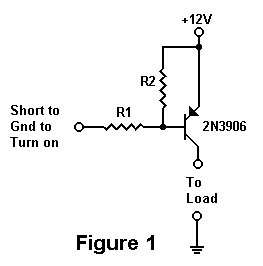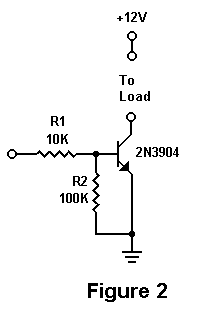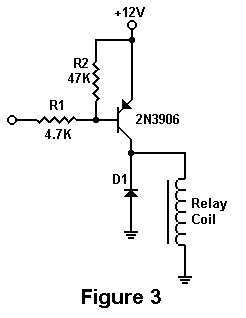Using Bipolar Transistors As Switches By Mike Martell N1HFX
 While transistors have many uses, one of the less known uses by amateurs is the ability for bipolar transistors to turn things on and off. While there are limitations as to what we can switch on and off, transistor switches offer lower cost and substantial reliability over conventional mechanical relays. In this article, we will review the basic principles for transistor switches using common bipolar transistors. The most commonly used transistor switch is the PNP variety shown in Figure 1. The secret to making a transistor switch work properly is to get the transistor in a saturation state. For this to happen we need to know the maximum load current for the device to be turned on and the minimum HFE of the transistor. For example, if we have a load that requires 100MA of current and a transistor with a minimum HFE of 100, we can then calculate the minimum base current required to saturate the transistor as follows: Minimum base current = 100 MA / 100 Minimum base current = 1 MA In actual practice, it is best to calculate about 30% more current than we will need to guarantee our transistor switch is always saturated.  In this case, we will use 1.3 MA. We must also select our supply voltage, so for this example we will use 12 volts. We can now calculate resistor R1 in the circuit as follows: Maximum Current Required = 100MA Supply Voltage = 12 VoltsR1 = Supply Voltage / ( Maximum Current Required / Minimum HFE * 1.3 ) R1 = 12 / (.1 / 100 * 1.3) R1 = 9230.7 or 10K for nearest standard value. Resistor R2 is not essential to this circuit but is generally used for stability and to insure that the transistor switch is completely turned off. This resistor insures that the base of the transistor does not go slightly negative which would cause a very small amount of collector current to flow. The value of this resistor is not critical but a value about 10 times R1 is normally chosen. For this circuit we will calculate R2 to be 10 times R1 as follows: R2 = 10 * 10000 R2 = 100K To turn on our transistor switch all that is needed is to short resistor R1 to the negative ground. While PNP transistors are normally used for a negative ground configuration, it is possible use a NPN transistor if a positive ground configuration is desired as indicated in Figure 2. The calculation of resistor values is identical to the PNP version. However, in the NPN transistor, R1 must be shorted to the positive end of the supply to turn the switch on. While our transistor switch can easily replace many mechanical relays, it does have a few drawbacks. The maximum design current must not be exceeded or the output voltage will be reduced. A short circuit of the output will overheat and destroy the transistor in many cases. Although the transistor is in saturation when turned on, about .3 volts is lost through the collector to the emitter of the transistor. We must also insure that the maximum power dissipation of the transistor is not exceeded. We can calculate the power dissipation by multiplying the current by .3 volts. In the case of 100 MA, the transistor must be able to withstand 30 milliwatts (.3 times .1).Transistor switches are used for a wide variety of applications. Many amateurs will notice that the circuit in Figure 1 is used as the PTT in many transmitter circuits. Transistor switches are commonly used to turn on transmitter circuits, LED�s, cooling fans and even relays. However, when using a transistor to turn on a relay coil, it is very important to use a 1N4001 diode reversed biased in parallel with the relay coil as in Figure 3. This is to prevent the kickback voltage in the reverse polarity from destroying the transistor. This reverse voltage occurs momentarily when the normal current stops flowing through the coil. It is good practice to always use a diode when turning on any inductive load. Transistor switches are often used to take the low-level output from logic circuits to turn on or turn off a particular device.The actual transistor used as a switch is not critical in these applications. Virtually any general purpose NPN or PNP transistor can be used as a switch. All that is needed is to know the minimum HFE and the power dissipation of the transistor. While most all transistors in a TO-92 case will have HFE�s of at least 100, many power transistors in TO-220 cases often have an HFE no greater than 25. It is essential to know the HFE or Beta of a transistor, so that we can have a large enough base current to achieve saturation. If a power transistor is used to turn on a high current device, it may be necessary to use another lower current transistor switch to drive a transistor switch used in a high current application. This is especially important when using a low current logic output from a CMOS IC. While there are a few applications where our transistor switches may not be suitable, it is usually a much more reliable and inexpensive alternative to using mechanical relays. These circuits are low in cost and offer ease of design for the radio amateur. DE N1HFX

### 2021-02-20 MIRROR COPY

This is a mirror copy only.
The original was originally found here
(dated: UNKNOWN (pre-2012)):
http://www.rason.org/Projects/transwit/transwit.htm